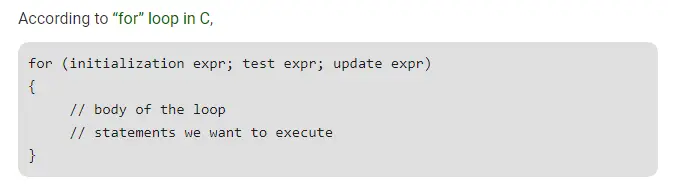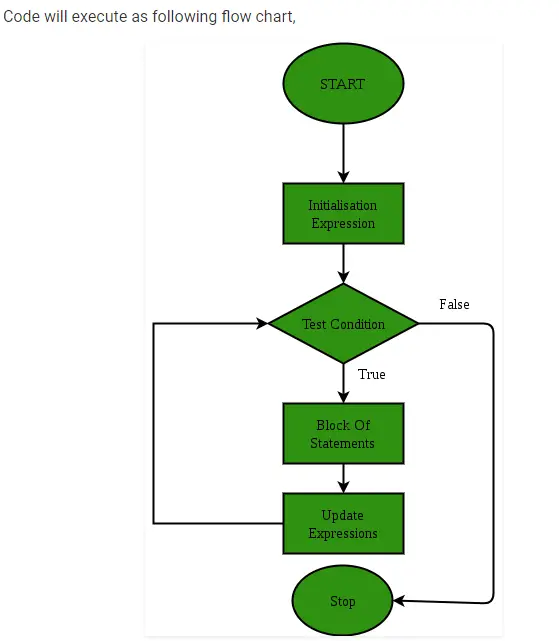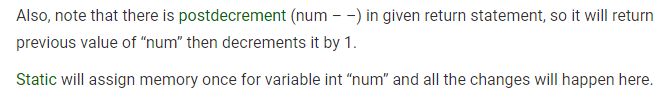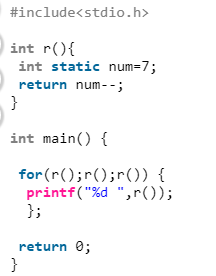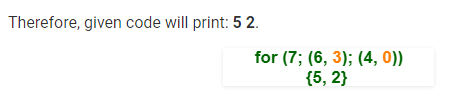# Gate CS-2019 Question Paper With Solutions

Q. 36 Consider the following C program:

``#include<stdio.h> ` ` ` `int` `r(){ ` ` ``int` `static` `num=7; ` ` ``return` `num--; ` `} ` ` ` `int` `main() { ` `     ` ` ``for``(r();r();r()) { ` `  ``printf``(``"%d "``,r()); ` `  ``}; ` `   ` ` ``return` `0; ` `} ``

Which one of the following values will be displayed on execution of the programs?

(A) 41

(B) 52

(C) 63

(D) 630